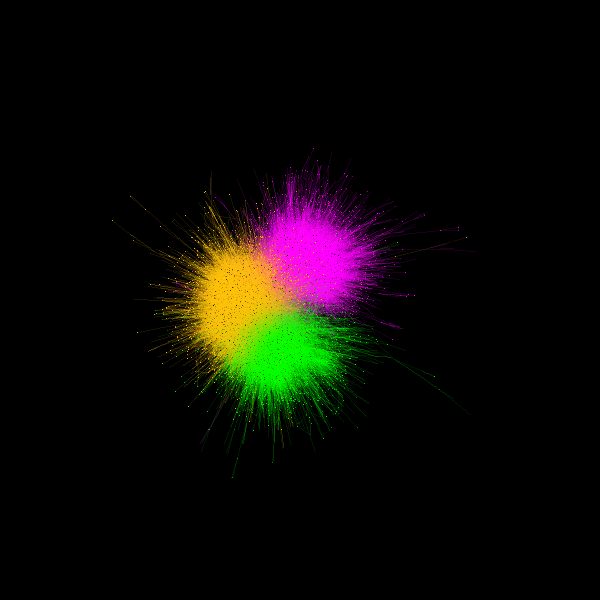# Visualize Interdisciplinary map of the journals network¶

The goal of this app is to show an overview of the journals network structure as a complex network. Each journal is shown as a node and their connections indicates a citation between two of them.

First, let’s import some useful functions

```from os.path import join as pjoin
from fury import actor, window, colormap as cmap
import numpy as np
```

```from fury.data.fetcher import fetch_viz_wiki_nw

files, folder = fetch_viz_wiki_nw()
categories_file, edges_file, positions_file = sorted(files.keys())
```

Out:

```Dataset is already in place. If you want to fetch it again please first remove the folder /Users/koudoro/.fury/examples/wiki_nw
```

```positions = np.loadtxt(pjoin(folder, positions_file))
```

We attribute a color to each category of our dataset which correspond to our nodes colors.

```category2index = {category: i
for i, category in enumerate(np.unique(categories))}

index2category = np.unique(categories)

categoryColors = cmap.distinguishable_colormap(nb_colors=len(index2category))

colors = np.array([categoryColors[category2index[category]]
for category in categories])
```

We define our node size

```radii = 1 + np.random.rand(len(positions))
```

Lets create our edges now. They will indicate a citation between two nodes. OF course, the colors of each edges will be an interpolation between the two node that it connects.

```edgesPositions = []
edgesColors = []
for source, target in edges:
edgesPositions.append(np.array([positions[source], positions[target]]))
edgesColors.append(np.array([colors[source], colors[target]]))

edgesPositions = np.array(edgesPositions)
edgesColors = np.average(np.array(edgesColors), axis=1)
```

Our data preparation is ready, it is time to visualize them all. We start to build 2 actors that we represent our data : sphere_actor for the nodes and lines_actor for the edges.

```sphere_actor = actor.sphere(centers=positions,
colors=colors,
theta=8,
phi=8,
)

lines_actor = actor.line(edgesPositions,
colors=edgesColors,
opacity=0.1,
)
```

All actors need to be added in a scene, so we build one and add our lines_actor and sphere_actor.

```scene = window.Scene()

```

The final step ! Visualize and save the result of our creation! Please, switch interactive variable to True if you want to visualize it.

```interactive = False

if interactive:
window.show(scene, size=(600, 600))

window.record(scene, out_path='journal_networks.png', size=(600, 600))
```This example can be improved by adding some interactivy with slider, picking, etc. Play with it, improve it!

Total running time of the script: ( 0 minutes 5.200 seconds)

Gallery generated by Sphinx-Gallery